# Contents

library(graper)
library(ggplot2)

# 1 Make example data with four groups

Create an example data set with 4 groups, 400 train + 100 test samples and 800 features.

set.seed(123)
data <- makeExampleData(n = 500, p=800, g=4,
pis=c(0.05, 0.1, 0.05, 0.1),
gammas=c(0.1, 0.1, 10, 10))
# training data set
Xtrain <- data$X[1:400, ] ytrain <- data$y[1:400]

# annotations of features to groups
annot <- data$annot # test data set Xtest <- data$X[401:500, ]
ytest <- data$y[401:500] # 2 Fit the model graper is the main function of this package, which allows to fit the proposed Bayesian models with different settings on the prior (by setting spikeslab to FALSE or TRUE) and the variational approximation (by setting factoriseQ to FALSE or TRUE). By default, the model is fit with a sparsity promoting spike-and-slab prior and a fully-factorised mean-field assumption. The parameter n_rep can be used to train multiple models with different random initializations. The best model is then chosen in terms of ELBO and returned by the function. th defines the threshold on the ELBO for convergence in the variational Bayes (VB) algorithm used for optimization. fit <- graper(Xtrain, ytrain, annot, n_rep=3, verbose=FALSE, th=0.001) ## Fitting a model with 4 groups, 400 samples and 800 features. ## Fitting with random init 1 ## ELB converged ## Fitting with random init 2 ## ELB converged ## Fitting with random init 3 ## ELB converged fit ## Sparse graper object for a linear regression model with 800 predictors in 4 groups. ## Group-wise shrinkage: ## 1 2 3 4 ## 0.22 0.07 8.25 7.13 ## Group-wise sparsity (1 = dense, 0 = sparse): ## 1 2 3 4 ## 0.06 0.14 0.04 0.1 # 3 Training diagnostics The ELBO monitors the convergence during training. plotELBO(fit)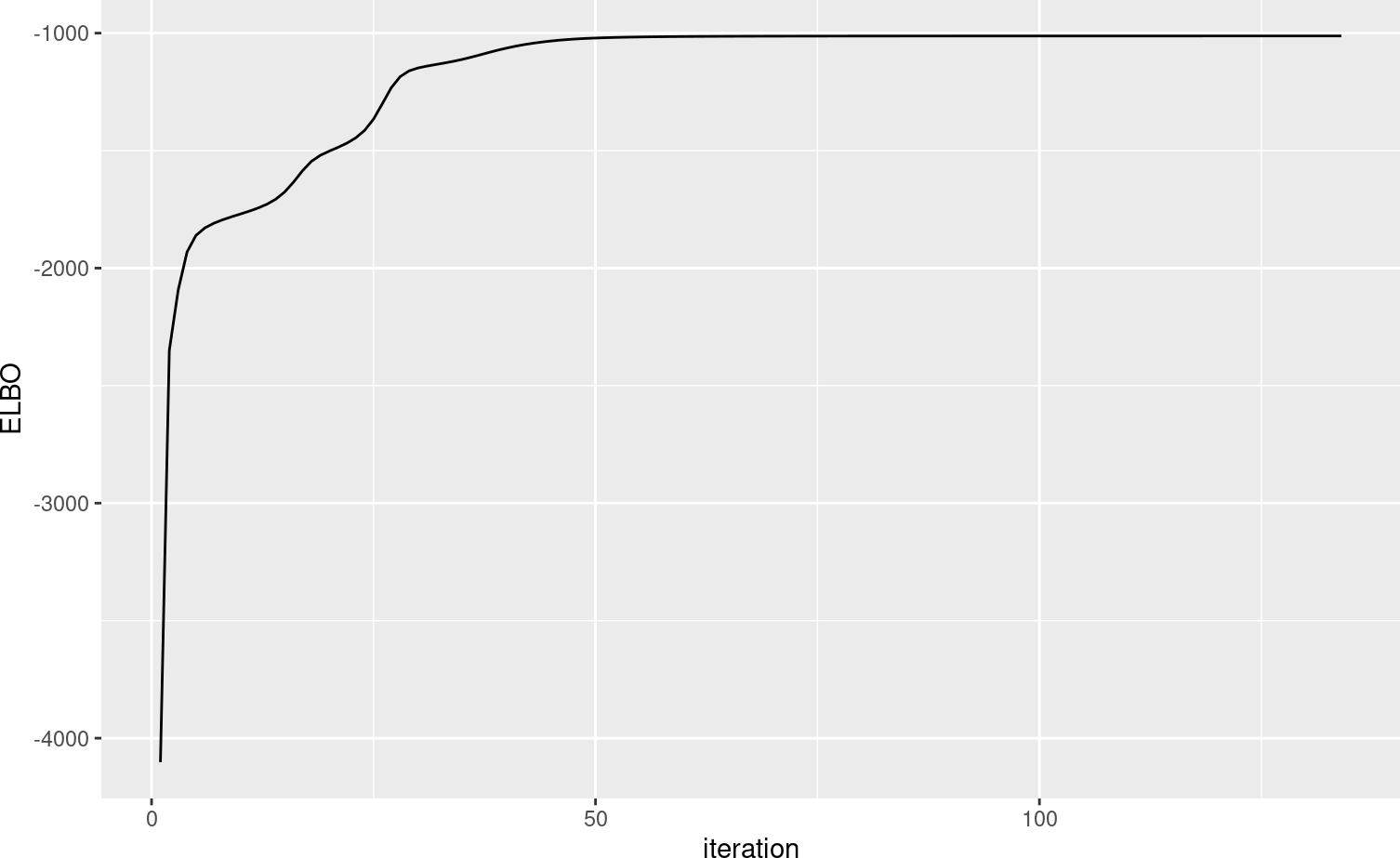# 4 Posterior distribtions The variational Bayes (VB) approach directly yields posterior distributions for each parameter. Note, however, that using VB these are often too concentrated and cannot be directly used for construction of confidence intervals etc. However, they can provide good point estimates. plotPosterior(fit, "gamma", gamma0=data$gammas, range=c(0, 20))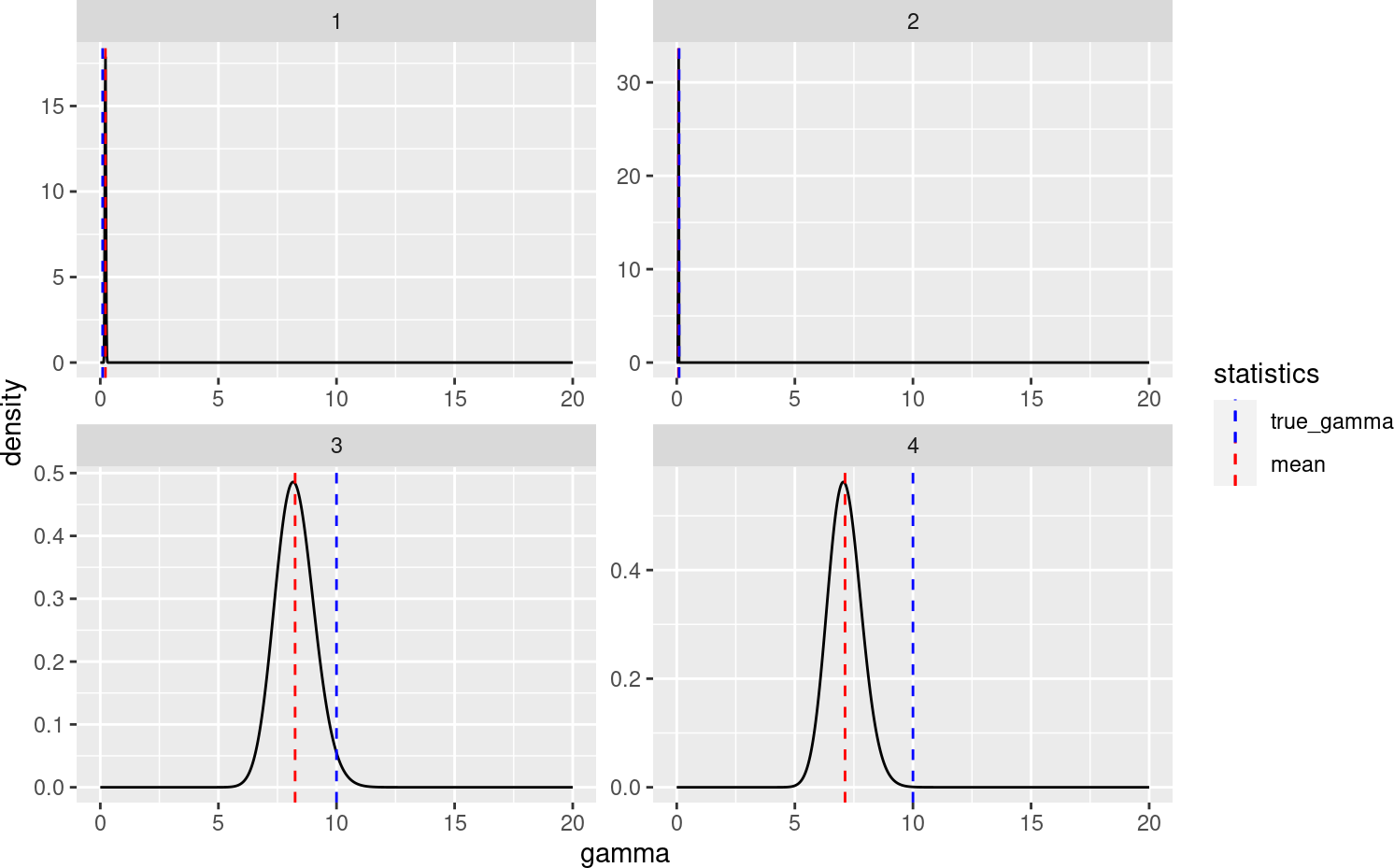plotPosterior(fit, "pi", pi0=data$pis)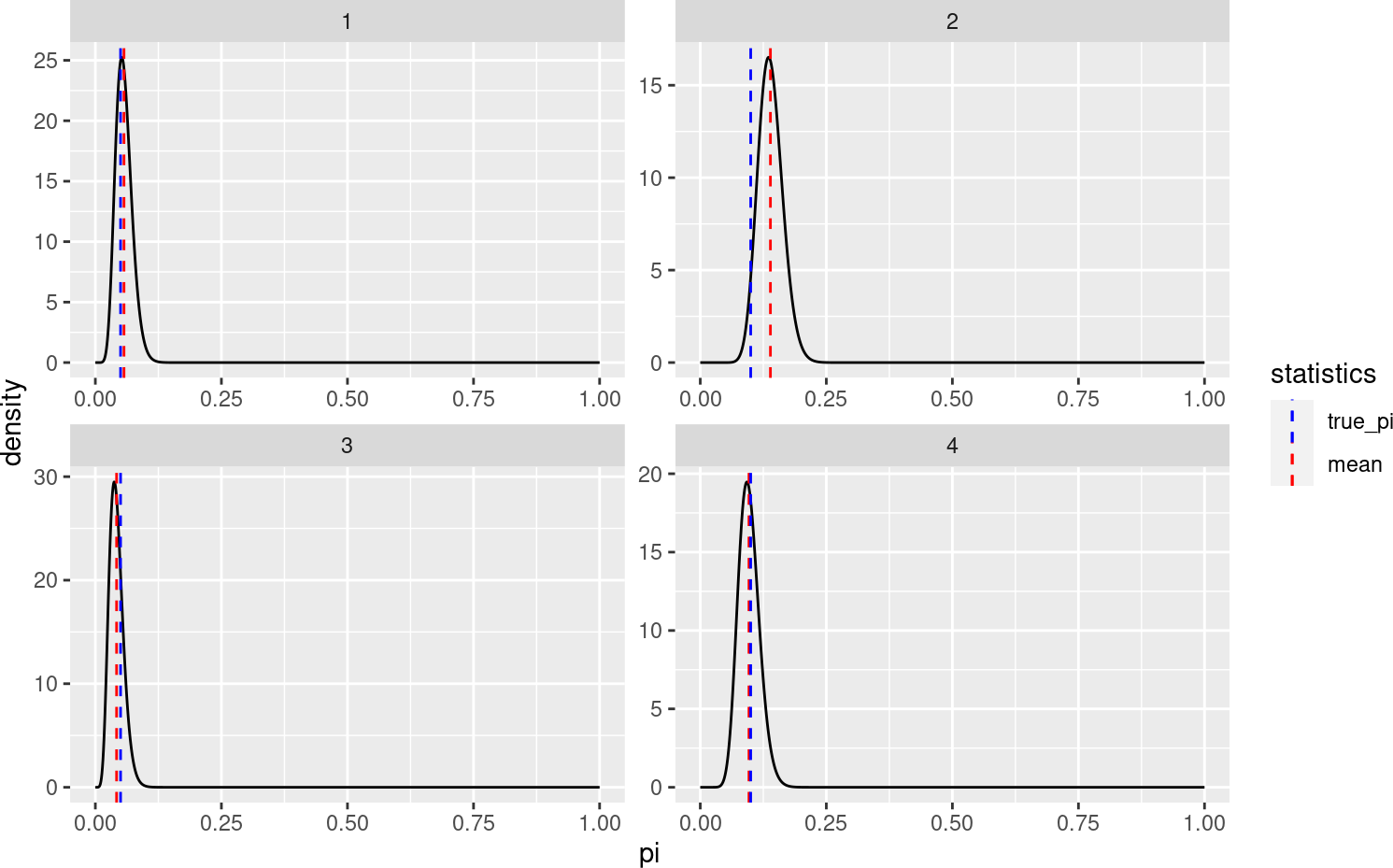# 5 Model coefficients and intercept The estimated coefficients and the intercept are contained in the result list. # get coefficients (without the intercept) beta <- coef(fit, include_intercept=FALSE) # beta <- fit$EW_beta

# plot estimated versus true beta
qplot(beta, data$beta) + coord_fixed() + theme_bw()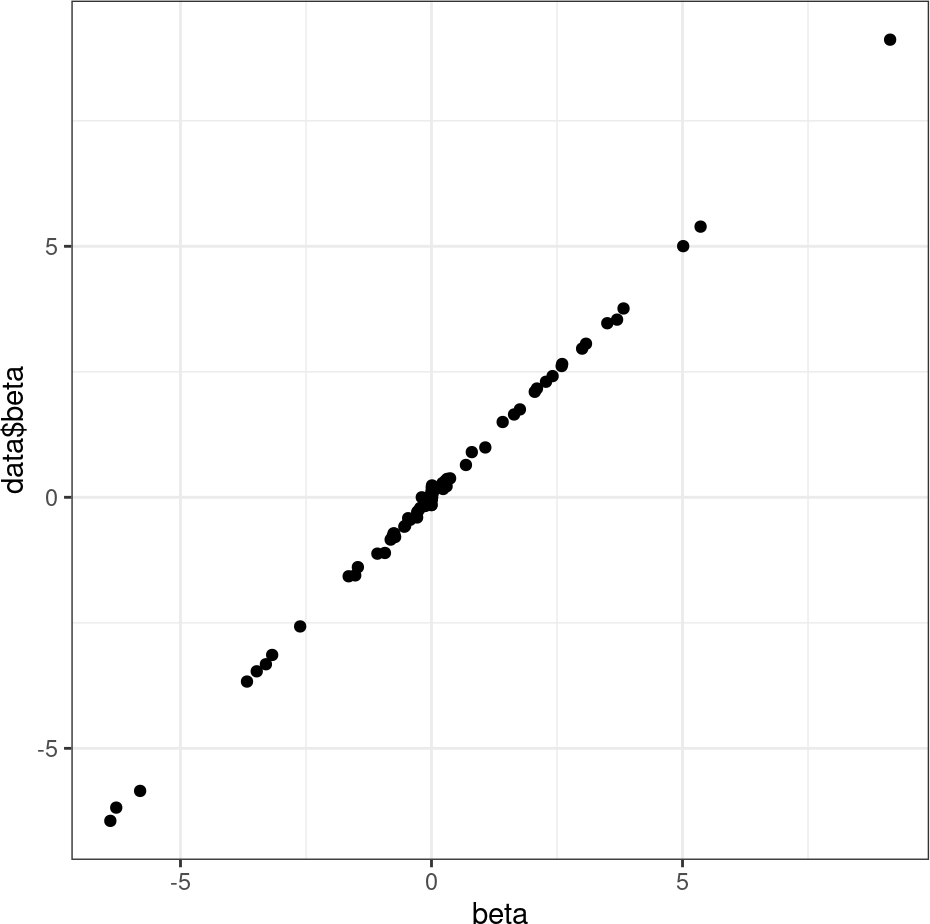# get intercept intercept <- fit$intercept

# 6 Posterior inclusion probabilities per feature

The estimated posterior inclusion probabilities per feature are contained in the result list and can also be accessed using getPIPs

# get estimated posterior inclusion probabilities per feature
pips <- getPIPs(fit)

# plot pips for zero versus non-zero features
df <- data.frame(pips = pips,
nonzero = data\$beta != 0)
ggplot(df, aes(x=nonzero, y=pips, col=nonzero)) +
geom_jitter(height=0, width=0.2) +
theme_bw() + ylab("Posterior inclusion probability")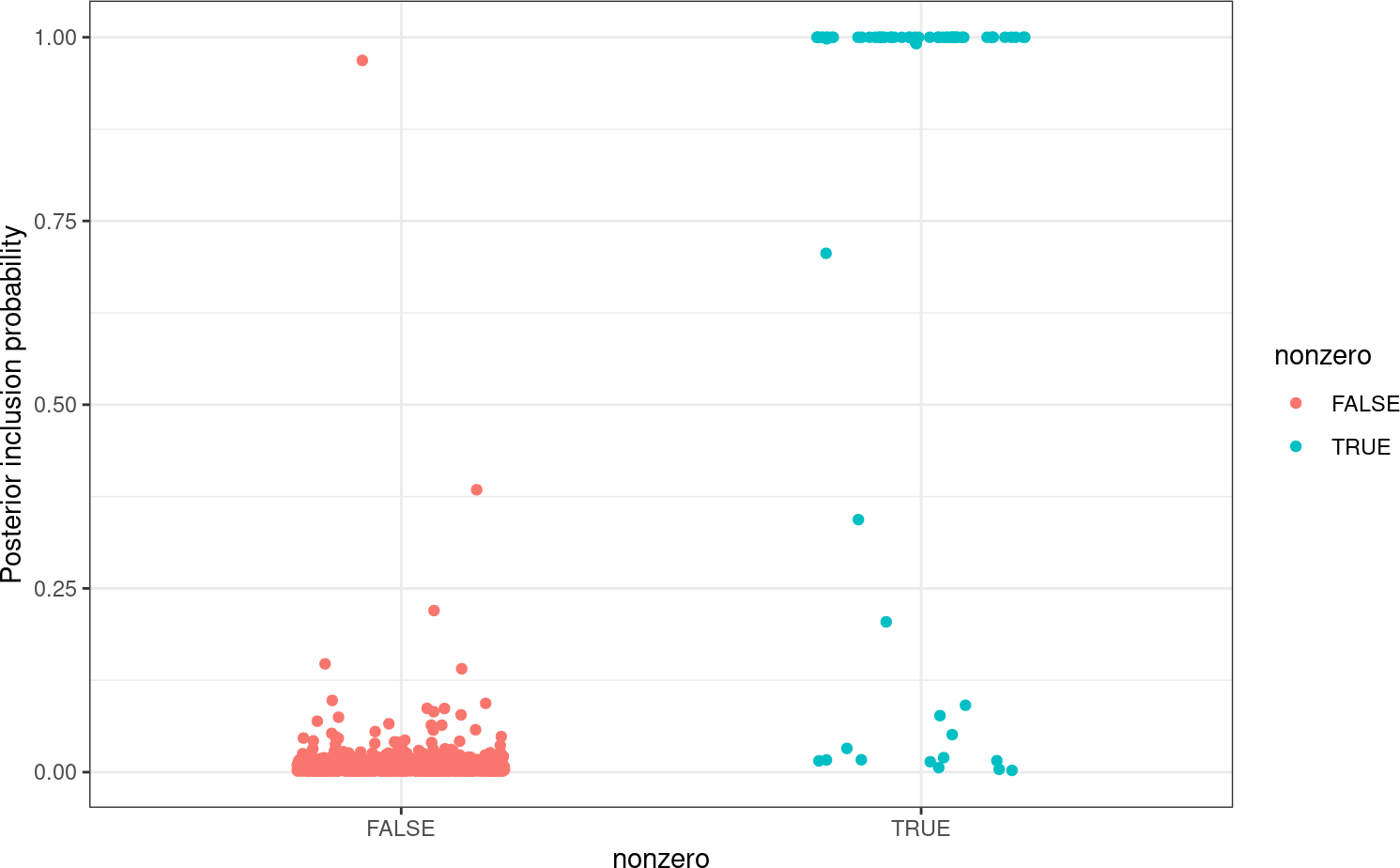# 7 Group-wise penalites

The function plotGroupPenalties can be used to plot the penalty factors and sparsity levels inferred for each feature group.

plotGroupPenalties(fit)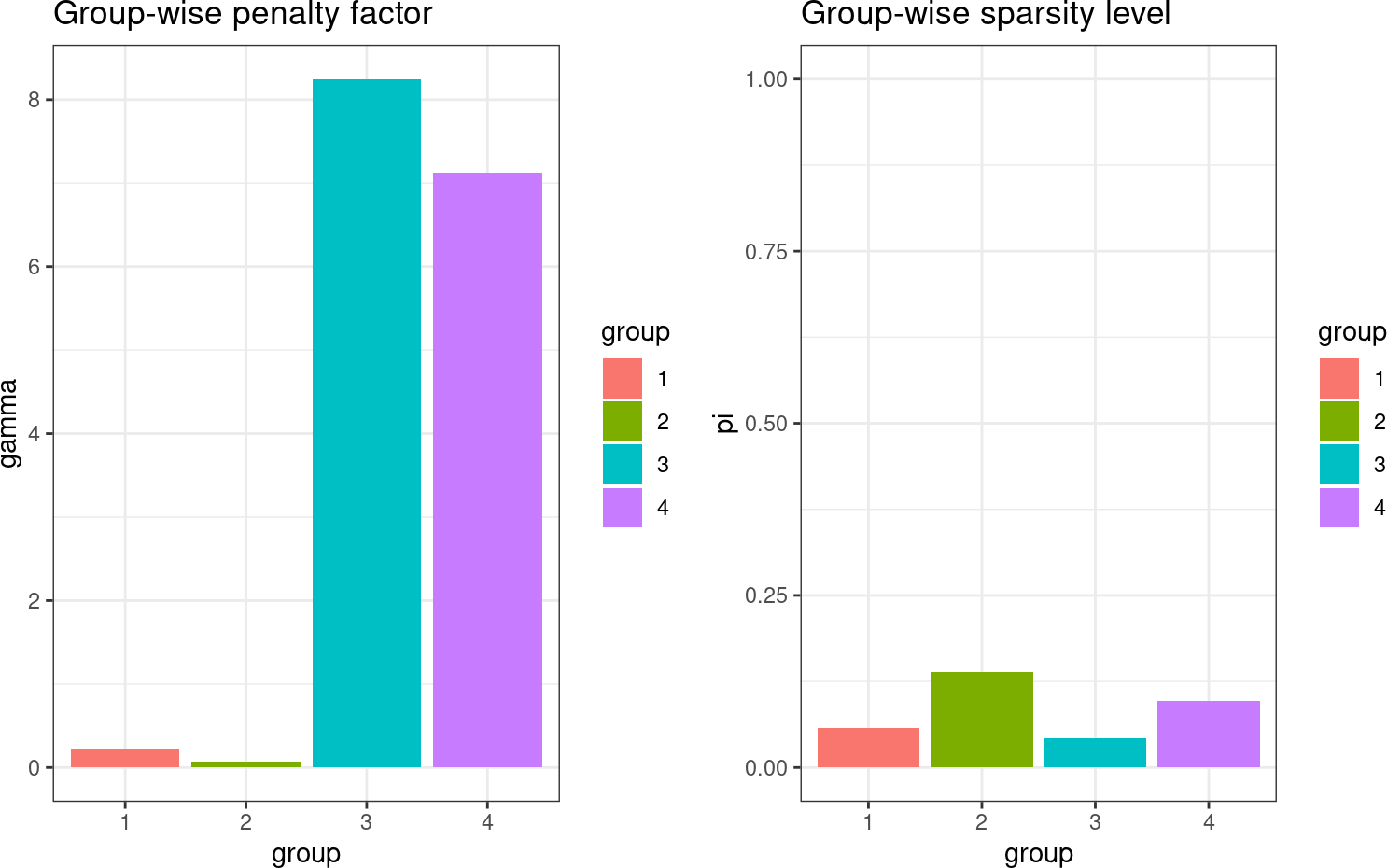# 8 Make predictions

The function predict can be used to make prediction on new data. Here, we illustrate its use by predicting the response on the test data defined above.

preds <- predict(fit, Xtest)
qplot(preds, ytest) +
coord_fixed() + theme_bw()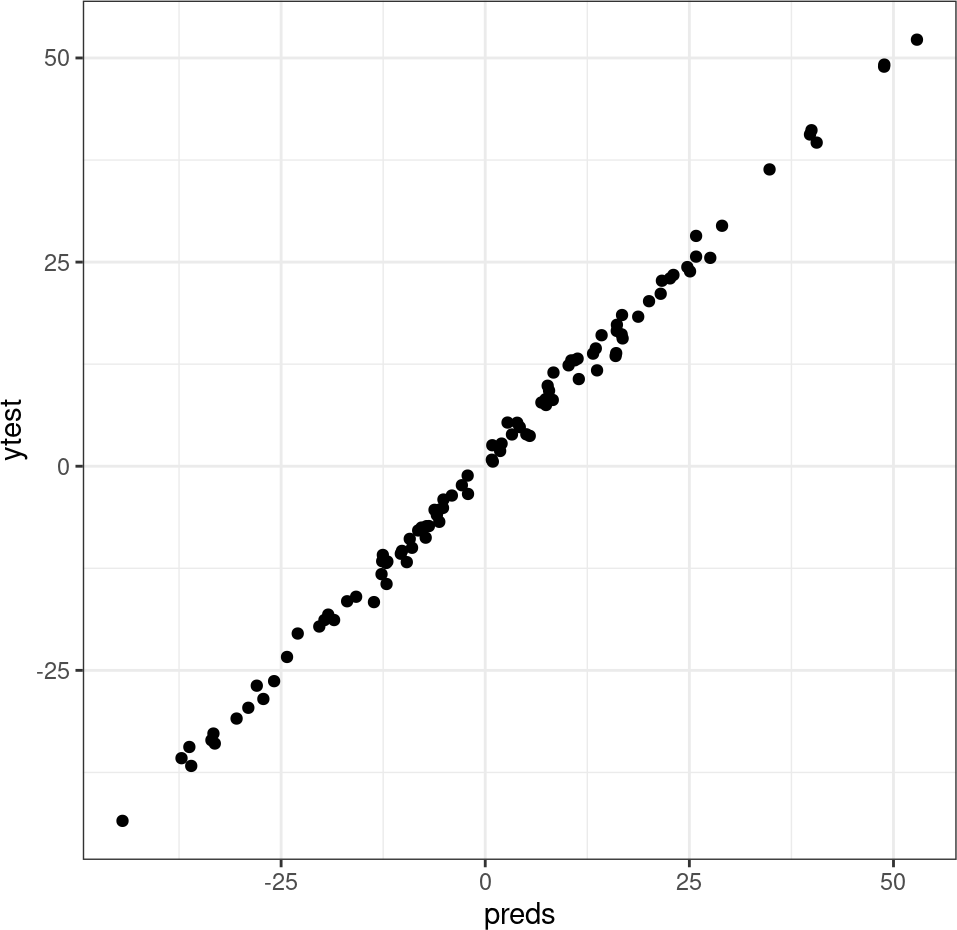#SessionInfo

sessionInfo()
## R version 4.2.0 RC (2022-04-19 r82224)
## Platform: x86_64-pc-linux-gnu (64-bit)
## Running under: Ubuntu 20.04.4 LTS
##
## Matrix products: default
## BLAS:   /home/biocbuild/bbs-3.15-bioc/R/lib/libRblas.so
## LAPACK: /home/biocbuild/bbs-3.15-bioc/R/lib/libRlapack.so
##
## locale:
##   LC_CTYPE=en_US.UTF-8       LC_NUMERIC=C
##   LC_TIME=en_GB              LC_COLLATE=C
##   LC_MONETARY=en_US.UTF-8    LC_MESSAGES=en_US.UTF-8
##   LC_PAPER=en_US.UTF-8       LC_NAME=C
##  LC_MEASUREMENT=en_US.UTF-8 LC_IDENTIFICATION=C
##
## attached base packages:
##  stats     graphics  grDevices utils     datasets  methods   base
##
## other attached packages:
##  ggplot2_3.3.5    graper_1.12.0    BiocStyle_2.24.0
##
## loaded via a namespace (and not attached):
##   tidyselect_1.1.2    xfun_0.30           bslib_0.3.1
##   purrr_0.3.4         lattice_0.20-45     colorspace_2.0-3
##   vctrs_0.4.1         generics_0.1.2      htmltools_0.5.2
##  yaml_2.3.5          utf8_1.2.2          rlang_1.0.2
##  jquerylib_0.1.4     pillar_1.7.0        glue_1.6.2
##  withr_2.5.0         DBI_1.1.2           matrixStats_0.62.0
##  lifecycle_1.0.1     stringr_1.4.0       munsell_0.5.0
##  gtable_0.3.0        evaluate_0.15       labeling_0.4.2
##  knitr_1.38          fastmap_1.1.0       fansi_1.0.3
##  highr_0.9           Rcpp_1.0.8.3        scales_1.2.0
##  BiocManager_1.30.17 magick_2.7.3        jsonlite_1.8.0
##  farver_2.1.0        digest_0.6.29       stringi_1.7.6
##  bookdown_0.26       dplyr_1.0.8         grid_4.2.0
##  cowplot_1.1.1       cli_3.3.0           tools_4.2.0
##  magrittr_2.0.3      sass_0.4.1          tibble_3.1.6
##  crayon_1.5.1        pkgconfig_2.0.3     ellipsis_0.3.2
##  Matrix_1.4-1        assertthat_0.2.1    rmarkdown_2.14
##  R6_2.5.1            compiler_4.2.0